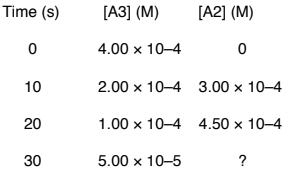# Problem: A concentration-time study of the gas phase reaction 2 A3 → 3 A2 produced the data in the table below.What is the average rate of decomposition of A3 in the time interval 20-30 seconds? Enter your answer in exponential format (example 1.23E-4) with two decimal places and no units.a. -6.2E-5b. -5.0E-6c. -4.3E-6d. +4.3E-6e. +3.2E-5

###### FREE Expert Solution

Determine the average rate of decomposition for A3 over a 20-30s time interval.

The rate of a chemical reaction is the speed at which the reactants are converted into products.

It is expressed as the change in concentrations of reactants or products over time.

The given reaction is:

2 A3 → 3 A2

84% (213 ratings)###### Problem Details

A concentration-time study of the gas phase reaction 2 A3 → 3 A2 produced the data in the table below.What is the average rate of decomposition of A3 in the time interval 20-30 seconds? Enter your answer in exponential format (example 1.23E-4) with two decimal places and no units.

a. -6.2E-5

b. -5.0E-6

c. -4.3E-6

d. +4.3E-6

e. +3.2E-5

Frequently Asked Questions

What scientific concept do you need to know in order to solve this problem?

Our tutors have indicated that to solve this problem you will need to apply the Average Rate of Reaction concept. You can view video lessons to learn Average Rate of Reaction. Or if you need more Average Rate of Reaction practice, you can also practice Average Rate of Reaction practice problems.

What is the difficulty of this problem?

Our tutors rated the difficulty ofA concentration-time study of the gas phase reaction 2 A3 → ...as medium difficulty.